Concurrent Statements 2 Concurrent statements are executed at

• Slides: 7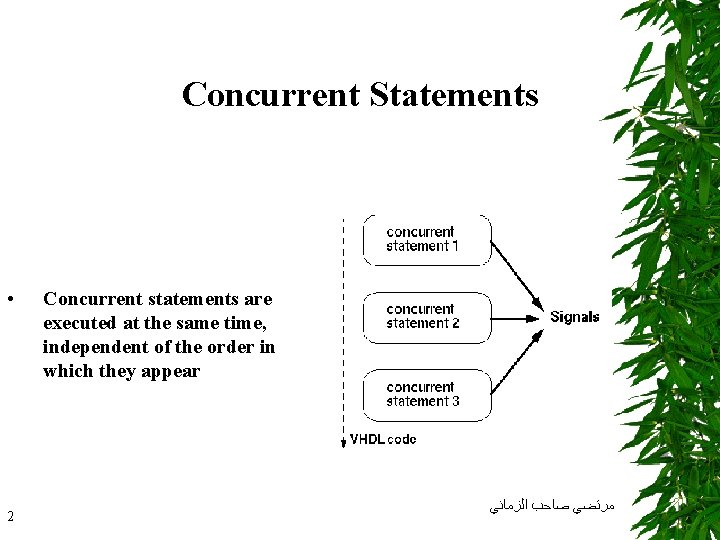Concurrent Statements • 2 Concurrent statements are executed at the same time, independent of the order in which they appear ﺍﻟﺰﻣﺎﻧﻲ ﺻﺎﺣﺐ ﻣﺮﺗﻀﻲConditional Signal Assignment TARGET <= VALUE_1 when CONDITION_1 else VALUE_2 when CONDITION_2 else . . . VALUE_n; • Condition is a Boolean expression • Mandatory else path, unless unconditional assignment • • conditions may overlap • priority Similar to if. . . , else constructs ﺩﻳگﺮ ﺑﺎﺭ ﺍﻧﺘﺴﺎﺏ ﺍﻳﻦ ﺩﻫﺪ ﺭﺥ ﺭﺍﺳﺖ ﺳﻤﺖ ﺳﻴگﻨﺎﻟﻬﺎﻱ ﺭﻭﻱ ﺗﻐﻴﻴﺮﻱ ﻫﺮگﺎﻩ • ( ﺍﺳﺖ ﻓﻌﺎﻝ ﺷﻮﺩ )ﻫﻤﻴﺸﻪ ﻣﻲ ﺍﺭﺯﻳﺎﺑﻲ 3 ﺍﻟﺰﻣﺎﻧﻲ ﺻﺎﺣﺐ ﻣﺮﺗﻀﻲ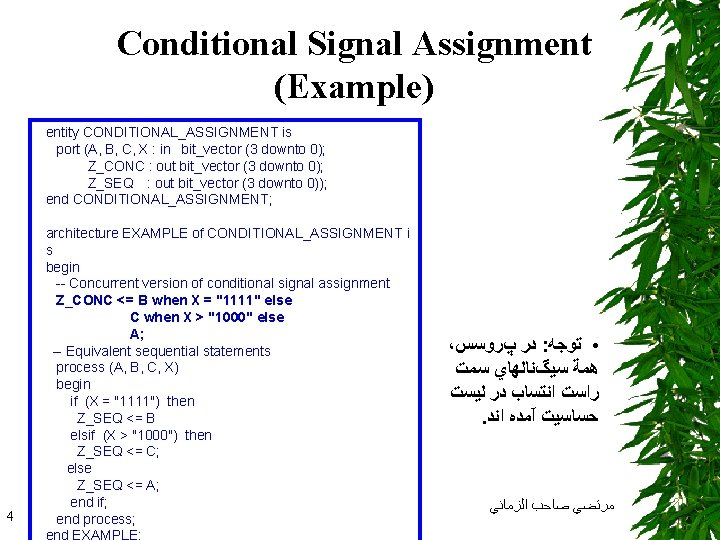Conditional Signal Assignment (Example) entity CONDITIONAL_ASSIGNMENT is port (A, B, C, X : in bit_vector (3 downto 0); Z_CONC : out bit_vector (3 downto 0); Z_SEQ : out bit_vector (3 downto 0)); end CONDITIONAL_ASSIGNMENT; 4 architecture EXAMPLE of CONDITIONAL_ASSIGNMENT i s begin -- Concurrent version of conditional signal assignment Z_CONC <= B when X = "1111" else C when X > "1000" else A; -- Equivalent sequential statements process (A, B, C, X) begin if (X = "1111") then Z_SEQ <= B elsif (X > "1000") then Z_SEQ <= C; else Z_SEQ <= A; end if; end process; ، پﺮﻭﺳﺲ ﺩﺭ : ﺗﻮﺟﻪ • ﺳﻤﺖ ﺳﻴگﻨﺎﻟﻬﺎﻱ ﻫﻤﺔ ﻟﻴﺴﺖ ﺩﺭ ﺍﻧﺘﺴﺎﺏ ﺭﺍﺳﺖ . ﺍﻧﺪ آﻤﺪﻩ ﺣﺴﺎﺳﻴﺖ ﺍﻟﺰﻣﺎﻧﻲ ﺻﺎﺣﺐ ﻣﺮﺗﻀﻲ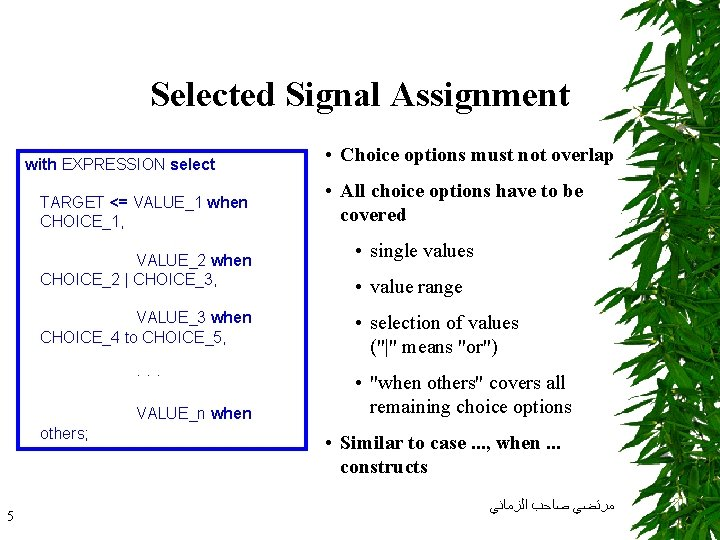Selected Signal Assignment with EXPRESSION select TARGET <= VALUE_1 when CHOICE_1, VALUE_2 when CHOICE_2 | CHOICE_3, • All choice options have to be covered • single values • value range VALUE_3 when CHOICE_4 to CHOICE_5, • selection of values ("|" means "or") · · · • "when others" covers all remaining choice options VALUE_n when others; 5 • Choice options must not overlap • Similar to case. . . , when. . . constructs ﺍﻟﺰﻣﺎﻧﻲ ﺻﺎﺣﺐ ﻣﺮﺗﻀﻲ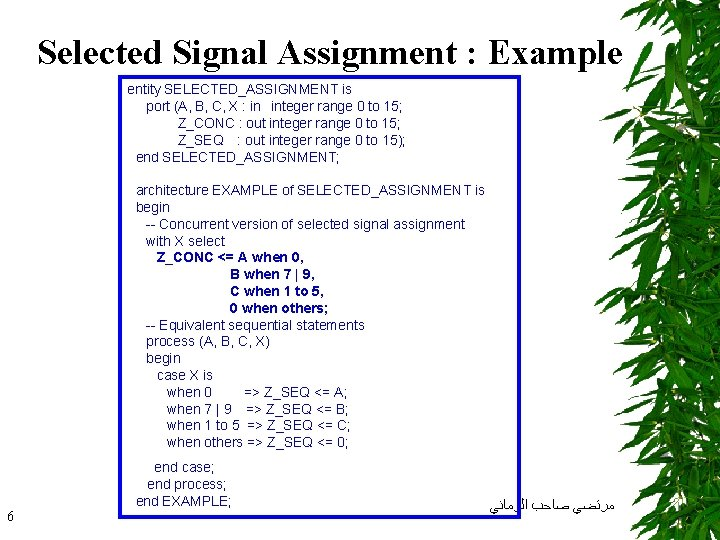Selected Signal Assignment : Example entity SELECTED_ASSIGNMENT is port (A, B, C, X : in integer range 0 to 15; Z_CONC : out integer range 0 to 15; Z_SEQ : out integer range 0 to 15); end SELECTED_ASSIGNMENT; architecture EXAMPLE of SELECTED_ASSIGNMENT is begin -- Concurrent version of selected signal assignment with X select Z_CONC <= A when 0, B when 7 | 9, C when 1 to 5, 0 when others; -- Equivalent sequential statements process (A, B, C, X) begin case X is when 0 => Z_SEQ <= A; when 7 | 9 => Z_SEQ <= B; when 1 to 5 => Z_SEQ <= C; when others => Z_SEQ <= 0; 6 end case; end process; end EXAMPLE; ﺍﻟﺰﻣﺎﻧﻲ ﺻﺎﺣﺐ ﻣﺮﺗﻀﻲ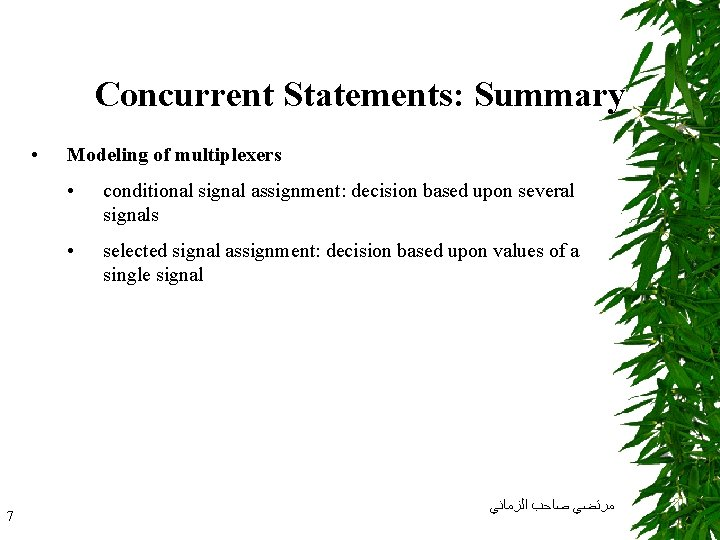Concurrent Statements: Summary • 7 Modeling of multiplexers • conditional signal assignment: decision based upon several signals • selected signal assignment: decision based upon values of a single signal ﺍﻟﺰﻣﺎﻧﻲ ﺻﺎﺣﺐ ﻣﺮﺗﻀﻲ